# Piecewise syndetic and van der Waerden

Joel Moreira has just proved that whenever the natural numbers are finitely coloured we can find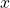and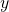such that,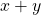and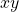are all the same colour.  He actually proves a much more general result via links to topological dynamics, but he includes a direct proof of this special case assuming only a consequence of van der Waerden’s theorem related to piecewise syndetic sets.  I want to record the details here so that I remember them in future.

## Syndetic, thick and piecewise syndetic

A set of natural numbers is thick if it contains (arbitrarily) long intervals, and syndetic if it has bounded gaps. It is piecewise syndetic if it has bounded gaps on long intervals (the same bound for each interval).

Piecewise syndetic sets are partition regular:

Claim. If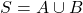is piecewise syndetic then either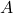or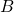is. (This extends to partitions into more parts by induction.)

Proof. Choose intervals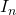of length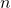on which the gaps in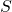are bounded by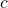. Suppose thatis not piecewise syndetic. Then for every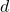there is an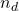such that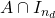has a gap more thanpoints wide. So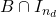contains an interval of lengthon which its gaps are bounded by.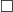It follows that, whenever the natural numbers are finitely coloured, one of the colour classes is piecewise syndetic. So to prove van der Waerden’s theorem it would suffice to show that every piecewise syndetic set has long arithmetic progressions. We’ll prove this apparent strengthening, assuming van der Waerden’s theorem itself.

Claim. Ifis piecewise syndetic, then it contains long APs.

Proof. Ifhas long intervals with gaps bounded by, then the union of thetranslates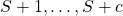ofcontains long intervals. Socan be viewed as a-colouring of a set of long intervals, hence one of them contains long APs by van der Waerden’s theorem. But they are all translates of, socontains long APs.In fact we can say much more: for every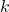there is asuch that the set of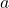such that the AP of lengthand common differencestarting atis contained inis piecewise syndetic. To see this, letbe a block of consecutive integers sufficiently long that whenever it is-coloured it contains an AP of length. Pack translates ofgreedily into the intervals in. For each translate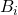ofthere is a triple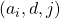such that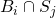contains the AP of lengthand common differencestarting at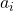. Now the set ofis piecewise syndetic (it has long intervals with gaps bounded by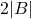). And there are only finitely many choices for(at most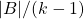) and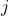(at most). So by the first claim there is some choice of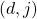such that the correspondingare piecewise syndetic. That is, the set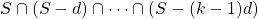is piecewise syndetic. This is Theorem 5.1 in Moreira’s paper, with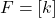and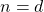. The case of generalfollows by taking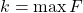.